# MySQL Tutorial: A Beginners Guide To Learn MySQLMySQL Tutorial: A Beginners Guide To Learn MySQL - Let's take a look at a very in-depth MySQL tutorial that explores all the operations and commands that you need to explore your databases...

MySQL Tutorial: A Beginners Guide To Learn MySQL - Let's take a look at a very in-depth MySQL tutorial that explores all the operations and commands that you need to explore your databases...

MySQL Tutorial is the second article in this blog series. In the previous article, What is MySQL, I introduced you to all the basic terminologies that you needed to understand before you get started with this relational database. In this blog of MySQL, you will be learning all the operations and commands that you need to explore your databases.

The topics covered in this article are mainly divided into 4 categories: DDL, DML, DC, and TCL.

• The DDL (Data Manipulation Language) consists of those commands which are used to define the database. Example: CREATE, DROP, ALTER, TRUNCATE, COMMENT, RENAME.
• The DML (Data Manipulation Language) commands deal with the manipulation of data present in the database. Example: SELECT, INSERT, UPDATE, DELETE.
• The DCL (Data Control Language) commands deal with the rights, permissions and other controls of the database system. Example: GRANT, INVOKE
• The TCL ( Transaction Control Language) consists of those commands which mainly deal with the transaction of the database.

Apart from the commands, following are the other topics covered in the blog:

• Different Types Of Keys In Database
• Constraints Used In Database
• Nested Queries
• Joins
• Set Operations

We are going to cover each of these categories one by one.

In this blog on MySQL Tutorial, I am going to consider the below database as an example, to show you how to write commands.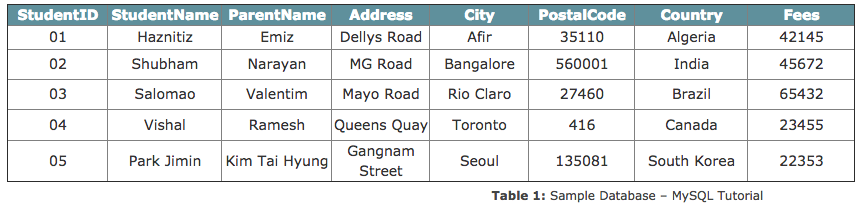So, let's get started now!

## MySQL Tutorial: Data Definition (DDL) Commands

This section consists of those commands, by which you can define your database. The commands are:

• CREATE
• ALTER
• DROP
• TRUNCATE
• RENAME

Now, before I start with the commands, let me just tell you the way to mention the comments in MySQL.

Like any other programming language, there are mainly two types of comments.

• *Single-Line Comments: *The single line comments start with ‘–‘. So, any text mentioned after — till the end of the line will be ignored by the compiler.

Example:

``````--Select all:
SELECT * FROM Students;
``````
• Multi-Line Comments: *The Multi-line comments start with / and end with /. So, any text mentioned between / and */ will be ignored by the compiler.

Example:

``````/*Select all the columns
of all the records
in the Students table:*/
SELECT * FROM Students;
``````

Now, that you know how to mention comments in MySQL, let's continue with the DDL commands.

## CREATE

The create statement is used to either create a schema, tables or an index.

### The 'CREATE SCHEMA' Statement

This statement is used to create a database.

Syntax:

``````CREATE SCHEMA Database_Name;
``````

Example:

``````CREATE SCHEMA StudentsInfo;
``````

### The 'CREATE TABLE' Statement

This statement is used to create a new table in a database.

##### Syntax:
``````CREATE TABLE table_name (
column1 datatype,
column2 datatype,
column3 datatype,
....
);
``````
##### Example:
``````CREATE TABLE Students
(
StudentID int,
StudentName varchar(255),
ParentName varchar(255),
PostalCode int,
City varchar(255)
);
``````

### The 'CREATE TABLE AS' Statement

This statement is used to create a new table from an existing table. So, this table gets the same column definitions as that of the existing table.

Syntax:

``````CREATE TABLE     SELECT     FROM new_table_name AScolumn1, column2,...existing_table_name     WHERE ....;
``````

Example:

``````CREATE TABLE ExampleTable AS
SELECT Studentname, Parentname
FROM Students;
``````

## ALTER

The ALTER command is used to add, modify or delete constraints or columns.

### The 'ALTER TABLE' Statement

This statement is used to either add, modify or delete constraints and columns from a table.

Syntax:

``````ALTER TABLE table_name
``````

Example:

``````ALTER TABLE Students
``````

## DROP

The DROP command is used to delete the database, tables, or columns.

### The 'DROP SCHEMA' Statement

This statement is used to drop the complete schema.

Syntax:

``````DROP SCHEMA schema_name;
``````

Example:

``````DROP SCHEMA StudentsInfo;
``````

### The 'DROP TABLE' Statement

This statement is used to drop the entire table with all its values.

Syntax:

``````DROP TABLE table_name;
``````

Example:

``````DROP TABLE table_name;
``````

## TRUNCATE

This statement is used to delete the data which is present inside a table, but the table doesn't get deleted.

Syntax:

``````TRUNCATE TABLE table_name;
``````

Example:

``````TRUNCATE TABLE Students;
``````

## RENAME

This statement is used to rename one or more tables.

Syntax:

``````RENAME TABLE
tbl_name TO new_tbl_name
[, tbl_name2 TO new_tbl_name2] ...
``````

Example:

``````RENAME Students TO Infostudents;
``````

Now, before I move into the further sections, let me tell you the various types of Keys and Constraints that you need to mention while manipulating the databases.

## Different Types of Keys in Database

There are mainly 5 types of Keys, that can be mentioned in the database.

• *Candidate Key: *The minimal set of attributes which can uniquely identify a tuple is known as a candidate key. A relation can hold more than a single candidate key, where the key is either a simple or composite key.
• *Super Key: *The set of attributes which can uniquely identify a tuple is known as Super Key. So, a candidate key is a superkey, but vice-versa isn't true.
• Primary Key: A set of attributes that can be used to uniquely identify every tuple is also a primary key. So, if there are 3-4 candidate keys present in a relationship, then out those, one can be chosen as a primary key.
• Alternate Key: **The candidate key other than the primary key is called as an alternate key.**
• *Foreign Key: *An attribute that can only take the values present as the values of some other attribute, is the foreign key to the attribute to which it refers.

## Constraints Used In Database

Refer to the image below are the constraints used in the database.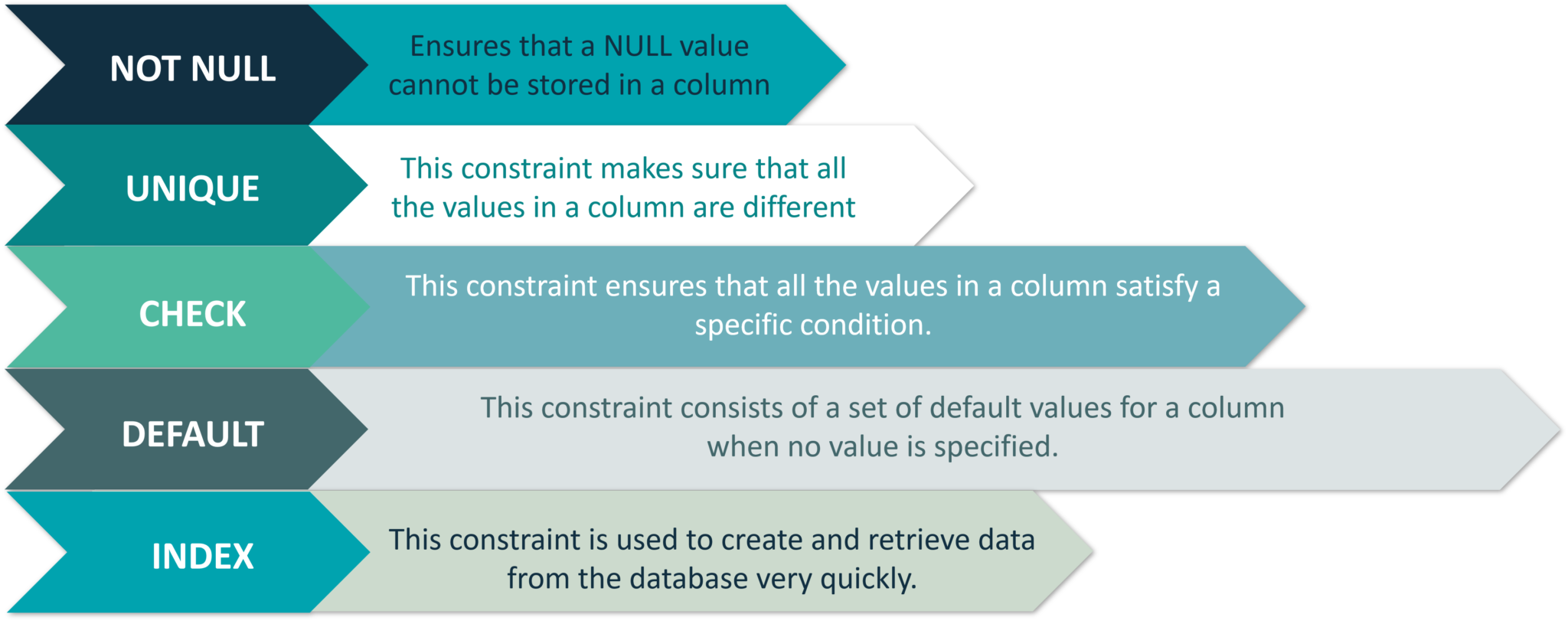Figure 1:* Constraints Used In Database: MySQL Tutorial*

Now that you know the various types of keys and constraints, let's move on to the next section i.e Data Manipulation Commands.

## Data Manipulation (DML) Commands

This section consists of those commands, by which you can manipulate your database. The commands are:

• USE
• INSERT
• UPDATE
• DELETE
• SELECT

Apart from these commands, there are also other manipulative operators/functions such as:

## USE

The USE statement is used to mention which database has to be used to perform all the operations.

Syntax:

``````USE Database_name;
``````

Example:

``````USE StudentsInfo;
``````

## INSERT

This statement is used to insert new records in a table.

The INSERT INTO statement can be written in the following two ways:

``````INSERT INTO table_name (column1, column2, column3, ...)
VALUES (value1, value2, value3, ...);
--You need not mention the column names
INSERT INTO table_name
VALUES (value1, value2, value3, ...);
``````

Example:

``````INSERT INTO Infostudents(StudentID, StudentName, ParentName, Address, City, PostalCode, Country)
VALUES ('06', 'Sanjana','Jagannath', 'Banjara Hills', 'Hyderabad', '500046', 'India');
INSERT INTO Infostudents
VALUES ('07', 'Shivantini','Praveen', 'Camel Street', 'Kolkata', '700096', 'India');
``````

## UPDATE

This statement is used to modify the existing records in a table.

Syntax:

``````UPDATE table_name
SET column1 = value1, column2 = value2, ...
WHERE condition;
``````

Example:

``````UPDATE Infostudents
SET StudentName = 'Alfred', City= 'Frankfurt'
WHERE StudentID = 1;
``````

## DELETE

This statement is used to delete existing records in a table.

Syntax:

``````DELETE FROM table_name
WHERE condition;
``````

Example:

``````DELETE FROM Infostudents
WHERE StudentName='Salomao';
``````

## SELECT

This statement is used to select data from a database and the data returned is stored in a result table, called the result-set.

The following are the two ways of using this statement:

Syntax:

``````SELECT column1, column2, ...
FROM table_name;
--(*) is used to select all from the table
SELECT * FROM table_name;
``````

Example:

``````SELECT StudentName, City FROM Infostudents;
SELECT * FROM Infostudents;
``````

Apart from the individual SELECT keyword, we will be also seeing the following statements, which are used with the SELECT keyword:

This statement is used to return only distinct or different values. So, if you have a table with duplicate values, then you can use this statement to list distinct values.

Syntax:

``````SELECT DISTINCT column1, column2, ...
FROM table_name;
``````

Example:

``````SELECT Country FROM Students;
``````

### The 'ORDER BY' Statement

This statement is used to sort the desired results in ascending or descending order. By default, the results would be sorted in ascending order. If you want the records in the result-set in descending order, then use the DESC keyword.

Syntax:

``````SELECT column1, column2, ...
FROM table_name
ORDER BY column1, column2, ... ASC|DESC;
``````

Example:

``````SELECT * FROM Infostudents
ORDER BY Country;
SELECT * FROM Infostudents
ORDER BY Country DESC;
SELECT * FROM Infostudents
ORDER BY Country, StudentName;
SELECT * FROM Infostudents
ORDER BY Country ASC, StudentName DESC;
``````

### The 'GROUP BY' Statement

This statement is used with the aggregate functions to group the result-set by one or more columns.

Syntax:

``````SELECT column_name(s)
FROM table_name
WHERE condition
GROUP BY column_name(s)
ORDER BY column_name(s);
``````

Example:

``````SELECT COUNT(StudentID), Country
FROM Infostudents
GROUP BY Country
ORDER BY COUNT(StudentID) DESC;
``````

### The 'HAVING' Clause Statement

Since the WHERE keyword cannot be used with aggregate functions, the HAVING clause was introduced.

Syntax:

``````SELECT column_name(s)
FROM table_name
WHERE condition
GROUP BY column_name(s)
HAVING condition
ORDER BY column_name(s);
``````

Example:

``````SELECT COUNT(StudentID), City
FROM Infostudents
GROUP BY City
HAVING COUNT(Fees) > 23000;
``````

## LOGICAL OPERATORS

This set of operators consists of logical operators such as AND/OR/NOT.

### AND OPERATOR

The AND operator is used to filter records that rely on more than one condition. This operator displays the records, which satisfy all the conditions separated by AND, and give the output TRUE.

Syntax:

``````ELECT column1, column2, ...
FROM table_name
WHERE condition1 AND condition2 AND condition3 ...;
``````

Example:

``````SELECT * FROM Infostudents
WHERE Country='Brazil' AND City='Rio Claro';
``````

### OR OPERATOR

The OR operator displays those records which satisfy any of the conditions separated by OR and gives the output TRUE.

Syntax:

``````SELECT column1, column2, ...
FROM table_name
WHERE condition1 OR condition2 OR condition3 ...;
``````

Example:

``````SELECT * FROM Infostudents
WHERE City='Toronto' OR City='Seoul';
``````

### NOT OPERATOR

This operator displays a record when the condition (s) is NOT TRUE.

Syntax:

``````SELECT column1, column2, ...
FROM table_name
WHERE NOT condition;
``````

Example:

``````SELECT * FROM Infostudents
WHERE NOT Country='India';
--You can also combine all the above three operators and write a query like this:
SELECT * FROM Infostudents
WHERE Country='India' AND (City='Bangalore' OR City='Canada');
``````

## Arithmetic, Bitwise, Comparison, and Compound Operators

Refer to the image below.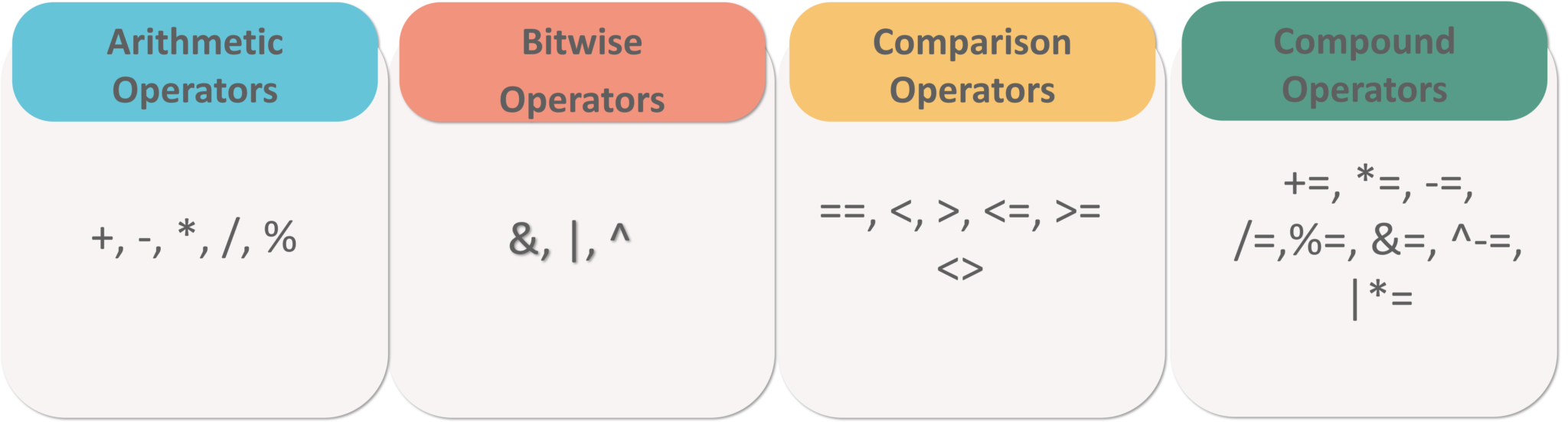Figure 2:* Arithmetic, Bitwise, Comparison & Compound Operators - MySQL Tutorial*

## Aggregate Functions

This section of functions include the following functions:

This function returns the smallest value of the selected column in a table.

Syntax:

``````SELECT MIN(column_name)
FROMtable_name
WHEREcondition;
``````

Example:

``````SELECT MIN(StudentID) AS SmallestID
FROM Infostudents;
``````

## MAX() Function

This function returns the largest value of the selected column in a table.

Syntax:

``````SELECT MAX(column_name)
FROM table_name
WHERE condition;
``````

Example:

``````SELECT MAX(Fees) AS SmallestFees
FROM Infostudents;
``````

## COUNT() Function

This function returns the number of rows that match the specified criteria.

Syntax:

``````SELECT COUNT(column_name)
FROM table_name
WHERE condition;
``````

Example:

``````SELECT COUNT(StudentID)
FROM Infostudents;
``````

## AVG() Function

This function returns the average value of a numeric column that you choose.

Syntax:

``````SELECT AVG(column_name)
FROM table_name
WHERE condition;
``````

Example:

``````SELECT AVG(Fees)
FROM Infostudents;
``````

## SUM() Function

This function returns the total sum of a numeric column that you choose.

Syntax:

``````SELECT SUM(column_name)
FROM table_name
WHERE condition;
``````

Example:

``````SELECT SUM(Fees)
FROM Infostudents;
``````

## SPECIAL OPERATORS

This section includes the following operators:

This operator is an inclusive operator, which selects values(numbers, texts or dates) within a given range.

Syntax:

``````SELECT column_name(s)
FROM table_name
WHERE column_name BETWEEN value1 AND value2;
``````

Example:

``````SELECT * FROM Infostudents
WHERE Fees BETWEEN 20000 AND 40000;
``````

### IS NULL Operator

Since it is not possible to test for the NULL values with the comparison operators(=, <, >), we can use IS NULL and IS NOT NULL operators instead.

Syntax:

``````--Syntax for IS NULL
SELECT column_names
FROM table_name
WHERE column_name IS NULL;
--Syntax for IS NOT NULL
SELECT column_names
FROM table_name
WHERE column_name IS NOT NULL;
``````

Example:

``````SELECT StudentName, ParentName, Address FROM Infostudents
SELECT StudentName, ParentName, Address FROM Infostudents
``````

### LIKE Operator

The mentioned below are the two wildcards that are used in conjunction with the LIKE operator:

• % — The percent sign represents zero, one, or multiple characters
• _ — The underscore represents a single character

Syntax:

``````SELECT column1, column2, ...
FROM table_name
WHERE column LIKE pattern;
``````

Refer to the following table for the various patterns that you can mention with LIKE operator.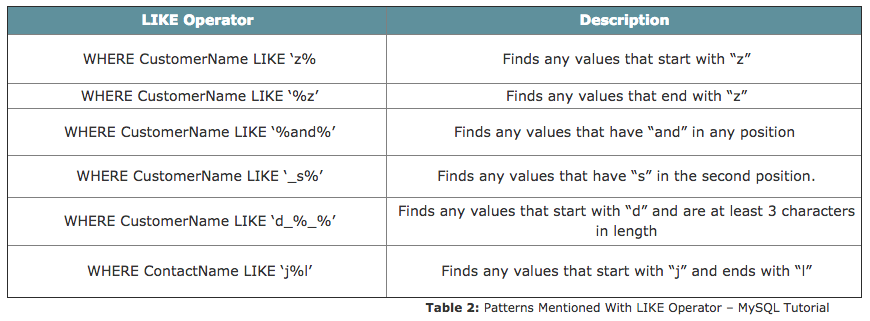Example:

``````SELECT * FROM Infostudents
WHERE StudentName LIKE 'S%';
``````

### IN Operator

This is a shorthand operator for multiple OR conditions which allows you to specify multiple values in a WHERE clause.

Syntax:

``````SELECT column_name(s)
FROM table_name
WHERE column_name IN (value1, value2, ...);
``````

Example:

``````SELECT * FROM Infostudents
WHERE Country IN ('Algeria', 'India', 'Brazil');
``````

Note: You can also use IN while writing Nested Queries. Consider the below syntax:

Syntax:

``````SELECT column_name(s)
FROM table_name
WHERE column_name IN (SELECT STATEMENT);
``````

### EXISTS Operator

This operator is used to test if a record exists or not.

Syntax:

``````SELECT column_name(s)
FROM table_name
WHERE EXISTS
(SELECT column_name FROM table_name WHERE condition);
``````

Example:

``````SELECT StudentName
FROM Infostudents
WHERE EXISTS (SELECT ParentName FROM Infostudents WHERE StudentId = 05 AND Price < 25000);
``````

### ALL Operator

This operator is used with a WHERE or HAVING clause and returns true if all of the subquery values meet the condition.

Syntax:

``````SELECT column_name(s)
FROM table_name
WHERE column_name operator ALL
(SELECT column_name FROM table_name WHERE condition);
``````

Example:

``````SELECT StudentName
FROM Infostudents
WHERE StudentID = ALL (SELECT StudentID FROM Infostudents WHERE Fees > 20000);
``````

### ANY Operator

Similar to the ALL operator, the ANY operator is also used with a WHERE or HAVING clause and returns true if any of the subquery values meet the condition.

Syntax:

``````SELECT column_name(s)
FROM table_name
WHERE column_name operator ANY
(SELECT column_name FROM table_name WHERE condition);
``````

Example:

``````SELECT StudentName
FROM Infostudents
WHERE StudentID = ANY (SELECT SttudentID FROM Infostudents WHERE Fees BETWEEN 22000 AND 23000);
``````

Now that I have told you a lot about DML commands, let me just tell you in short about Nested Queries, Joins, and Set Operations.

## Nested Queries

Nested queries are those queries which have an outer query and inner subquery. So, basically, the subquery is a query which is nested within another query such as SELECT, INSERT, UPDATE or DELETE. Refer to the image below: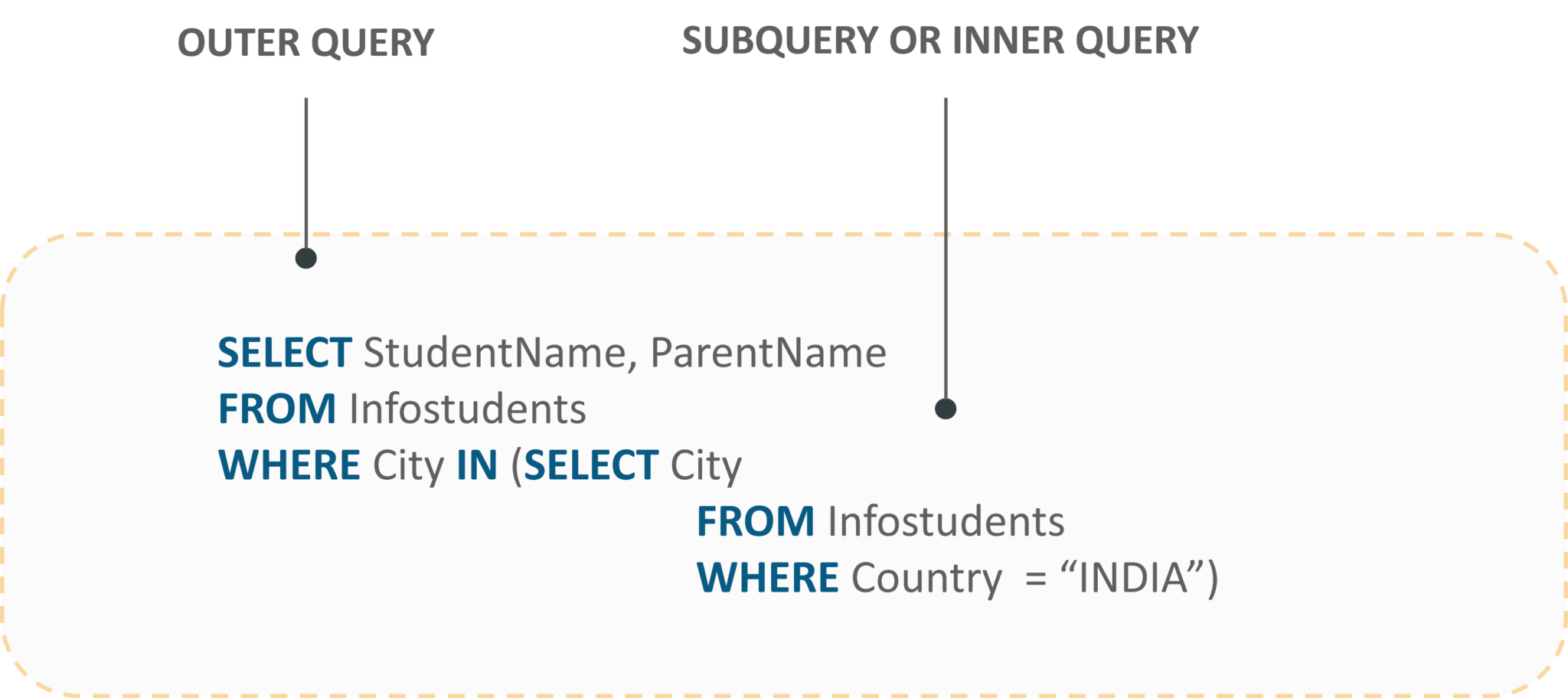Fig 3:* Representation Of Nested Queries - MySQL Tutorial*

JOINS are used to combine rows from two or more tables, based on a related column between those tables. The following are the types of joins:

• INNER JOIN: This join returns those records which have matching values in both the tables.
• FULL JOIN: This join returns all those records which either have a match in the left or the right table.
• LEFT JOIN: This join returns records from the left table, and also those records which satisfy the condition from the right table.
• RIGHT JOIN: This join returns records from the right table, and also those records which satisfy the condition from the left table.

Refer to the image below.Fig 4:* Representation Of Joins: MySQL Tutorial*

Let's consider the below table apart from the Infostudents table, to understand the syntax of joins.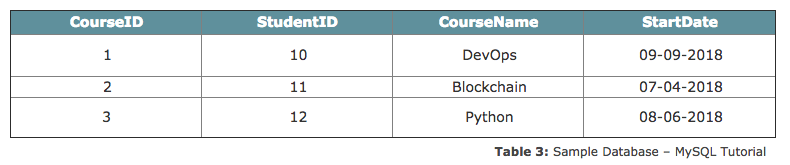### INNER JOIN

Syntax:

``````SELECT column_name(s)
FROM table1
INNER JOIN table2 ON table1.column_name = table2.column_name;
``````

Example:

``````SELECT Courses.CourseID, Infostudents.StudentName
FROM Courses
INNER JOIN Infostudents ON Courses.StudentID = Infostudents.StudentID;
``````

### FULL JOIN

Syntax:

``````SELECT column_name(s)
FROM table1
FULL OUTER JOIN table2 ON table1.column_name = table2.column_name;
``````

Example:

``````SELECT Infostudents.StudentName, Courses.CourseID
FROM Infostudents
FULL OUTER JOIN Orders ON Infostudents.StudentID=Orders.StudentID
ORDER BY Infostudents.StudentName;
``````

### LEFT JOIN

Syntax

``````SELECT column_name(s)
FROM table1
LEFT JOIN table2 ON table1.column_name = table2.column_name;
``````

Example:

``````SELECT Infostudents.StudentName, Courses.CourseID
FROM Infostudents
LEFT JOIN Courses ON Infostudents.CustomerID = Courses.StudentID
ORDER BY Infostudents.StudentName;
``````

### RIGHT JOIN

Syntax:

``````SELECT column_name(s)
FROM table1
RIGHT JOIN table2 ON table1.column_name = table2.column_name;
``````

Example:

``````SELECT Courses.CourseID
FROM Courses
RIGHT JOIN Infostudents ON Courses.StudentID = Infostudents.StudentID
ORDER BY Courses.CourseID;
``````

## Set Operations

There are mainly three set operations: UNION, INTERSECT, SET DIFFERENCE. You can refer to the image below to understand the set operations in SQL.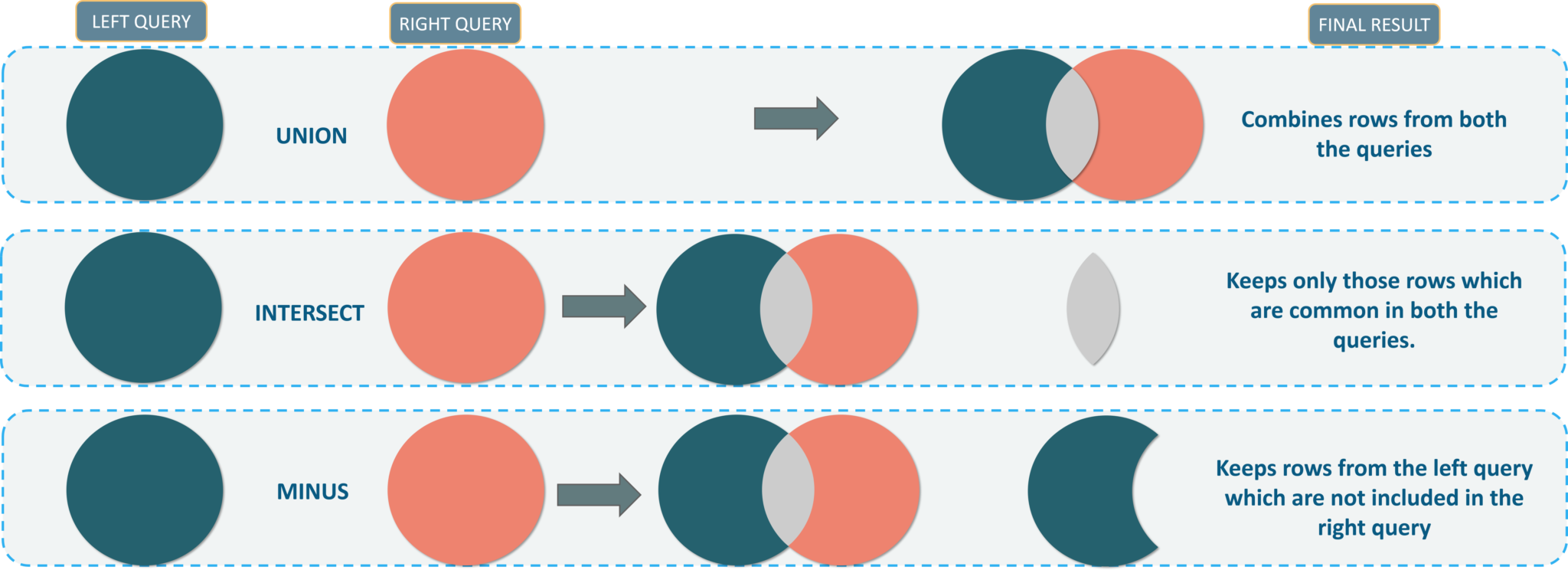Now, that you guys know the DML commadsn. Let’s move onto our next section and see the DCL commands.

## Data Control (DCL) Commands

This section consists of those commands which are used to control privileges in the database. The commands are:

This command is used to provide user access privileges or other privileges for the database.

Syntax:

``````GRANT privileges ON object TO user;
``````

Example:

``````GRANT CREATE ANY TABLE TO localhost;
``````

### REVOKE

This command is used to withdraw user’s access privileges given by using the GRANT command.

Syntax:

``````REVOKE privileges ON object FROM user;
``````

Example:

``````REVOKE INSERT ON *.* FROM Infostudents;
``````

Now, let’s move on to the last section of this blog i.e. the TCL Commands.

## Transaction Control (TCL) Commands

This section of commands mainly deals with the transaction of the database. The commands are:

This command saves all the transactions to the database since the last COMMIT or ROLLBACK command.

Syntax:

``````COMMIT;
``````

Example:

``````DELETE FROM Infostudents WHERE Fees = 42145;

COMMIT;
``````

### ROLLBACK

This command is used to undo transactions since the last COMMIT or ROLLBACK command was issued.

Syntax:

``````ROLLBACK;
``````

Example:

``````DELETE FROM Infostudents WHERE Fees = 42145;

ROLLBACK;
``````

### SAVEPOINT

This command creates points within the groups of transactions in which to ROLLBACK. So, with this command, you can simply roll the transaction back to a certain point without rolling back the entire transaction.

Syntax:

``````SAVEPOINT SAVEPOINT_NAME; --Syntax for saving the SAVEPOINT

ROLLBACK TO SAVEPOINT_NAME; --Syntax for rolling back to the Savepoint command
``````

Example:

``````SAVEPOINT SP1;

DELETE FROM Infostudents WHERE Fees = 42145;

SAVEPOINT SP2;
``````

### RELEASE SAVEPOINT

You can use this command to remove a SAVEPOINT that you have created.

Syntax:

``````RELEASE SAVEPOINT SAVEPOINT_NAME;
``````

Example:

``````RELEASE SAVEPOINT SP2;
``````

### SET TRANSACTION

This command gives a name to the transaction.

Syntax:

``````SET TRANSACTION [ READ WRITE | READ ONLY ];
``````

## Best MySQL DigitalOcean Performance – ScaleGrid vs. DigitalOcean Managed Databases

Compare ScaleGrid MySQL vs. DigitalOcean Managed Databases - See which offers the best MySQL throughput, latency, and pricing on DigitalOcean across workloads.

With the rapid development in technology, the old ways to do business have changed completely. A lot more advanced and developed ways are ...

## Hire Dedicated eCommerce Web Developers | Top eCommerce Web Designers

Build your eCommerce project by hiring our expert eCommerce Website developers. Our Dedicated Web Designers develop powerful & robust website in a short span of time.